# Areas in Polar Coordinates

 Exam 1 Wed Feb 1 7:00pm in Pepper Canyon 109 (not 106!! different class there!) Office hours: 2:45pm-4:15pm Next: Complex numbers (appendix G); complex exponentials (supplement, which is freely available online). We will not do arc length. People were most confused last time by plotting curves in polar coordinates. (1) it is tedious, but easier if you do a few and know what they look like (just plot some points and see); there's not much to it, except plug in values and see what you get, and (2) can sometimes convert to a curve in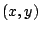coordinates, which might be easier. GOAL for today: Integration in the context of polar coordinates. Get much better at working with polar coordinates!

Example 4.2.1 (From Stewart.)   Find the area enclosed by one leaf of the four-leaved rose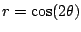.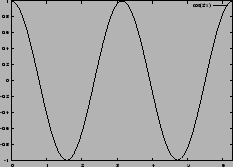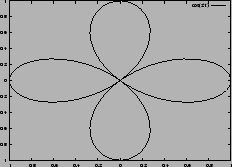To find the area using the methods we know so far, we would need to find a function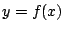that gives the height of the leaf.

Multiplying both sides of the equationby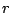yields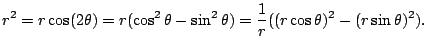Because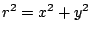and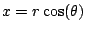and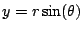, we have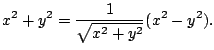Solving for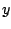is a crazy mess, and then integrating? It seems impossible!

But it isn't... if we remember the basic idea of calculus: subdivide and take a limit.

[[Draw a section of a curvefor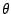in some interval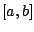, and shade in the area of the arc.]]

Remark 4.2.2   We will almost never talk about angles in degrees--we'll almost always use radians.

We know how to compute the area of a sector, i.e., piece of a circle with angle. [[draw picture]]. This is the basic polar region. The area is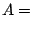(fraction of the circle)(area of circle)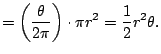We now imitate what we did before with Riemann sums. We chop up, approximate, and take a limit. Break the interval of angles from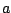to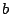into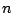subintervals. Choose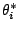in each interval. The area of each slice is approximately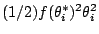. ThusArea of the shaded region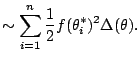Taking the limit, we see that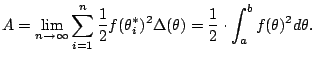Amazing! By understanding the definition of Riemann sum, we've derived a formula for areas swept out by a polar graph. But does it work in practice? Let's revisit our clover leaf.

Subsections
William Stein 2006-03-15